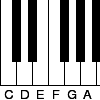#### You may also like### Pent

The diagram shows a regular pentagon with sides of unit length. Find all the angles in the diagram. Prove that the quadrilateral shown in red is a rhombus.### Tuning and Ratio

Why is the modern piano tuned using an equal tempered scale and what has this got to do with logarithms?### Rarity

Show that it is rare for a ratio of ratios to be rational.

# Reductant Ratios

##### Age 16 to 18 Challenge Level:

A very uniform grade of iron ore is known to be entirely formed from a mixture of different oxides of iron (iron oxide FeO, magnetite Fe$_3$O$_4$ and hematite Fe$_2$O$_3$), although their ratios are not known.

$1$g of this mixture is fully reduced, leaving $0.748$g of pure iron.

Must all three of the oxides indicated be present in the mixture?

Is there a smallest or largest amount of each oxide present?

What possibilities for the percentage composition of the mixture by mass does the experiment indicate?

Can you create a yield of iron which would prove the absence of at least one of the oxides of iron from the ore?